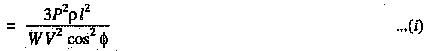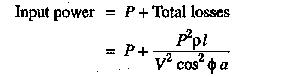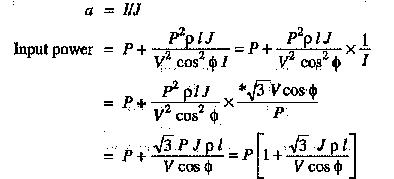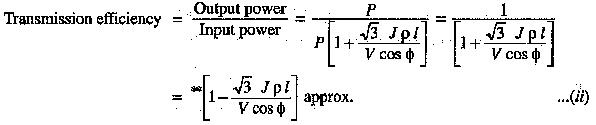# Advantages of High Transmission Voltage

## Advantages of High Transmission Voltage:

The Advantages of High Transmission Voltage at high voltages due to the following reasons :

(i) Reduces volume of conductor material: Consider the transmission of electric power by a three-phase line.

Let

P = power transmitted in watts

V = line voltage in volts

cos Φ = power factor of the load

l = length of the line in metres

R = resistance per conductor in ohms

ρ = resistivity of conductor material

a = area of X-section of conductorIt is clear from exp. (i) that for given values of P,l,ρ and W, the volume of conductor material required is inversely proportional to the square of transmission voltage and power factor. In other words, the greater the transmission voltage, the lesser is the conductor material required.

(ii) Increases transmission efficiencyAssuming J to be the current density of the conductor, thenAs J, ρ and l are constants, therefore, transmission efficiency increases when the line voltage is increased.

(iii) Decreases percentage line dropAs J, ρ and l are constants, therefore, percentage line drop decreases when the transmission voltage increases.

Limitations of high transmission voltage:

From the above discussion, it might appear advisable to use the highest possible voltage for transmission of power in a bid to save conductor material. However, it must be realised that high transmission voltage results in

• the increased cost of insulating the conductors
• the increased cost of transformers, switchgear and other terminal apparatus.

Therefore, there is a limit to the higher transmission voltage which can be economically employed in a particular case. This limit is reached when the saving in cost of conductor material due to higher voltage is offset by the increased cost of insulation, transformer, switchgear etc. Hence, the choice of proper transmission voltage is essentially a question of economics.